• 多元贝叶斯回归模型框架下绘制成本_效果可接受曲线_
• 多媒体 设置 要从此存储库自动检索并安装最新版本的R软件包，请运行 devtools :: install_github( " stephenslab/mvsusieR " ) 快速开始 是显示mvsusieR实际操作的快速文档。 有关更多文档和示例，请访问： : ...
• Wishart分布变量与多个x2分布变量之积具有相同的统计分布这一关系，以及正定阵之间变换的Jacobian行列式，推导了多元质量特性过程能力指数的后验分布，并据此构造了参数共轭先验分布下的多元贝叶斯过程能力指数及其...
• 1. 什么是朴素贝叶斯朴素贝叶斯是一种构建分类器，该分类器基于一个理论：所有朴素贝叶斯分类器都假定样本每个特征与其他特征都不相关2. 朴素贝叶斯概率模型概率模型分类器是一个条件概率模型：（独立的类别特征C有...
1. 什么是朴素贝叶斯朴素贝叶斯是一种构建分类器，该分类器基于一个理论：所有朴素贝叶斯分类器都假定样本每个特征与其他特征都不相关2. 朴素贝叶斯概率模型概率模型分类器是一个条件概率模型：（独立的类别特征C有若干类别，条件依赖于若干特征变量F1,F2,...,Fn）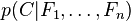贝叶斯定理：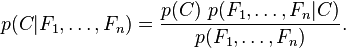我们可以看到分母并不依赖于C，而且特征Fn的概率是给定的，分母可以认为是一个常数。这样分子就等价于联合分布模型， 使用链式法则，可将该式写成条件概率的形式，如下所示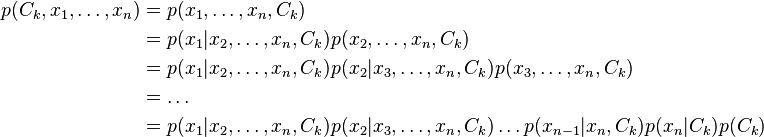假设每个特征Fi对于其他特征Fj是条件独立的。这就意味着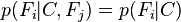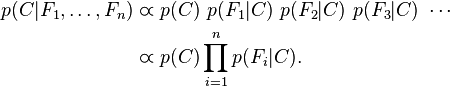3. 多元朴素贝叶斯p(X|Ck)表示事件i发生的概率，特征向量X=(x1,x2,...,xn)，其中xi表示事件i在特定的对象中被观察到的次数。 X的似然函数如下所示：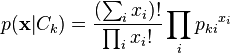多元朴素贝叶斯分类器：使用对数转化成线性分类器。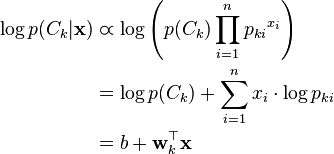如果一个事件在特征向量i中没有出现过，那么i的特征值概率将为0，而0的对数是无效的，需要对每个小类样本概率进行修正，以保证不会出现有为0的概率出现，需要加上平滑系数，常用到的平滑就是加1平滑（也称拉普拉斯平滑）。多元朴素贝叶斯常用于文档的归类分析4. Spark实现多元朴素贝叶斯4.1 计算事件i的特征向量的次数要实现多元朴素贝叶斯模型，首先要归并同一事件i的特征向量的次数，那么我们很容易想到函数AggregateByKey，事件i作为key进行归并    val aggregated = dataset.select(col($(labelCol)), w, col($(featuresCol))).rdd
.map { row => (row.getDouble(0), (row.getDouble(1), row.getAs[Vector](2)))
}.aggregateByKey[(Double, DenseVector)]((0.0, Vectors.zeros(numFeatures).toDense))(
seqOp = {
case ((weightSum: Double, featureSum: DenseVector), (weight, features)) =>
requireValues(features)
BLAS.axpy(weight, features, featureSum)
(weightSum + weight, featureSum)
},
combOp = {
case ((weightSum1, featureSum1), (weightSum2, featureSum2)) =>
BLAS.axpy(1.0, featureSum2, featureSum1)
(weightSum1 + weightSum2, featureSum1)
}).collect().sortBy(_._1)DataSet的支持：在Spark 2.1后部分mlib库对dataset进行支持，在这种情况下需要把原来的LabeledPoint进行转化成DataSetWeight 权重支持：你可以定义每一个特征纬度的权重，特征纬度的权重最后会影响log(P(Ck))的值，默认的权重设置为1seqOp：在同一个Partation中的合并操作 combOp: 不同的Partition 的最后合并操作 4.2 计算事件i的概率    val piLogDenom = math.log(numDocuments + numLabels * lambda)
var i = 0
aggregated.foreach { case (label, (n, sumTermFreqs)) =>
labelArray(i) = label
piArray(i) = math.log(n + lambda) - piLogDenom
......
}
}计算事件I的概率对数：log(P|Ck) = math.log((n+lambda)/(numDocuments+numLabels*lamdba))假如：有两个事件A,B,特征向量是x1,x2,x3数据集合如下：A 1 2 3A 3 4 1B 3 2 4B 5 7 1计算log(P|A)n: 就是事件A的数量 2（权重是1的情况下）numDocuments: 就是总数据集合的数量 4numLabels: 就是合并后剩下的数据集合的数量 2，也就是事件的数量(A,B)lambda:就是为了防止为0的平滑系数 4.3 计算事件i的每个特征的概率 val thetaLogDenom = $(modelType) match { case Multinomial => math.log(sumTermFreqs.values.sum + numFeatures * lambda) case _ => // This should never happen. throw new UnknownError(s"Invalid modelType:${\$(modelType)}.")
}
var j = 0
while (j < numFeatures) {
thetaArray(i * numFeatures + j) = math.log(sumTermFreqs(j) + lambda) - thetaLogDenom
j += 1
}计算每个特征的概率对数：log(Pxi)=math.log((sumtermfreqs(i)+lambda)/(sumtermfreqs.values.sum+numFeatures*lamdba))沿用上面的例子：sumtermfreqs(i):就是每个特征值xi在事件A下的数量sumtermfreqs.values.sum 就是所有特征值x1...xn在事件A下的数量和numfeatures: 就是总的特征向量的数量n4.4 构建矩阵和向量生成模型向量：事件A,B的概率向量举证：事件A,B的每一个特征的概率，构成一个以特征数量为行，以事件为列的密度矩阵，保存到朴素贝叶斯模型中去 val pi = Vectors.dense(piArray)
val theta = new DenseMatrix(numLabels, numFeatures, thetaArray, true)
new NaiveBayesModel(uid, pi, theta).setOldLabels(labelArray)4.5 如何进行分类按照多元朴素贝叶斯计算模型计算log(P(Ck))+sum(xi*log(Pki))为归为某个事件的概率log(p(Ck))和log(Pki)都已经在前面计算好了，唯一要做的是将要用于分类的数据*log(Pki)并且求和，加上log(Pck)就可以了，会不会很奇怪为什么是求和而不是求乘法，通常贝叶斯公式都是乘法，因为这里是求了对数，所以乘法变成了加法如何分类？MAP决策准则：选出算出最大的概率所属的分类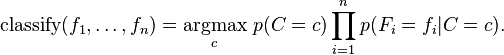4.6 如何判定模型有效使用常用的交叉验证方式，将已经分类好的数据集合随机分成测试数据和训练数据，使用训练数据进行训练计算概率矩阵，使用测试数据进行预测分类，计算预测的准确率，设定自己定义的准确率，如果达到就判定训练的模型有效。    val Array(training, test) = data.randomSplit(Array(0.6, 0.4))

val model = NaiveBayes.train(training, lambda = 1.0, modelType = "multinomial")

val predictionAndLabel = test.map(p => (model.predict(p.features), p.label))
val accuracy = 1.0 * predictionAndLabel.filter(x => x._1 == x._2).count() / test.count()
展开全文• 本篇博客所写的算法对应于吴恩达教授的机器学习教程里的多元伯努利事件模型的朴素贝叶斯多元伯努利事件模型的Python代码 #!/usr/bin/env python # -*- coding: utf-8 -*- # @Time : 2018/9/415:55 # @...
前言

本篇博客所写的算法对应于吴恩达教授的机器学习教程里的多元伯努利事件模型的朴素贝叶斯。

多元伯努利事件模型的Python代码

#!/usr/bin/env python
# -*- coding: utf-8 -*-
# @Time    : 2018/9/415:55
# @Author  : DaiPuWei
# E-Mail   : 771830171@qq.com
# @Site    : 北教25实验室
# @File    : NaiveBayes.py
# @Software: PyCharm

import numpy as np

"""
这份朴素贝叶斯的代码对应于吴恩达机器学习笔记中多元伯努利模型
即每组词向量的每一维的取值为0或1。
"""

class NaiveBayes_Bernoulli(object):
def __init__(self,Train_Data,Train_Label):
"""
这厮朴素贝叶斯的构造函数
:param Train_Data: 训练数据
:param Train_Label:训练标签
"""
# 生成字典
self.VocabularyList = self.CreateVocabularyList(Train_Data)
self.Train_Label = Train_Label
self.Train_Data = Train_Data
# 文本数据向量化
self.Train_Data_Vector = []
for data in self.Train_Data:
self.Train_Data_Vector.append(self.Word2Vector(data,self.VocabularyList))
self.Train_Data_Vector = np.array(self.Train_Data_Vector)
# 初始化朴素贝叶斯的相关参数
self.py1 = 0                                # p(y=1)
self.py0 = 0                                # p(y=0)
col = len(self.VocabularyList)
self.pj_y1 = np.array(*col)              # p(x_j|y=1)
self.pj_y0 = np.array(*col)              # p(x_j|y=0)

def CreateVocabularyList(self,dataset):
"""
这是利用数据集构造词汇表（字典）的函数
:param dataset: 数据集
"""
# 初始化字典,
VocabularyList = set([])
for data in dataset:
for _data in data:
# 集合内不会包含重复元素
return list(VocabularyList)

def Word2Vector(self,input_data,VocabularyList):
"""
这是将一组数据向量化的函数
:param input_data: 输入数据
:param VocabularyList: 字典
"""
_data = *len(VocabularyList)
# enumerate(input_data)返回文字与对应下标的元组
for (index,data) in enumerate(input_data):
if data in VocabularyList:
_data[index] = 1
return _data

def Train(self):
"""
这是朴素贝叶斯分类器的训练函数，这里利用拉普拉斯平滑对结果进行修正
"""
# 计算标签为0和1的个数
_py0 = self.Train_Label == 0
_py1 = self.Train_Label == 1
num_label0 = len(_py0)
num_label1 = len(_py1)
# 计算p(y=1)和p(y=0)
self.py1 = num_label1/float(num_label0+num_label1)
self.py0 = num_label0/float(num_label1+num_label0)
# 计算p(x_j|y=1)和p(x_j|y=0)，加入了拉普拉斯平滑来修正结果
p1_num = 2.0
p0_num = 2.0
for (index,data) in enumerate(self.Train_Data_Vector):
# np.sum(data)为每数据中1的总和
if self.Train_Label[index] == 1:
self.pj_y1 += data
p1_num += np.sum(data)
else:
self.pj_y0 += data
p0_num += np.sum(data)
self.pj_y1 = (self.pj_y1+1)*1.0/p1_num
self.pj_y0 = (self.pj_y0+1)*1.0/p0_num

def predict(self,test_data):
"""
这是对一组数据进行预测的函数
:param test_data: 一组测试函数,类型为np.array
"""
"""
由于p(x_j|y=1)和p(x_j|y=0)数组中的每一项大小过小，从而导致连乘过后
导致数值下溢，因此我们采取折回策略，首先将其取对数后再做指数运算，获得近似结果。
"""
_p0 = np.exp(np.sum(test_data * np.log(self.pj_y0)) + np.log(self.py0))
_p1 = np.exp(np.sum(test_data * np.log(self.pj_y1)) + np.log(self.py1))
px = _p0+_p1
p0 = float(_p0)/float(px)
p1 = float(_p1)/float(px)
print(p0)
print(p1)
if p1 >= p0:
return 1
else:
return 0

def Test(self,Test_Data):
# 数据向量化
Test_Data_Vector = []
for test_data in Test_Data:
Test_Data_Vector.append(self.Word2Vector(test_data,self.VocabularyList))
Test_Predict_Label = []
for test_data in Test_Data_Vector:
Test_Predict_Label.append(self.predict(test_data))
return Test_Predict_Label

测试代码

下面的代码的数据来自于《机器学习实战》。

#!/usr/bin/env python
# -*- coding: utf-8 -*-
# @Time    : 2018/9/910:23
# @Author  : DaiPuWei
# E-Mail   : 771830171@qq.com
# @Site    : 北教25实验室
# @File    : Bernoulli_demo.py
# @Software: PyCharm

import numpy as np
from NaiveBayes.NaiveBayes_Bernoulli import NaiveBayes_Bernoulli

def run_main():
"""
这是主函数
"""
# 初始化训练数据与标签
traindata = np.array([['my', 'dog', 'has', 'flea', 'problems', 'help', 'please'],
['maybe', 'not', 'take', 'him', 'to', 'dog', 'park', 'stupid'],
['my', 'dalmation', 'is', 'so', 'cute', 'I', 'love', 'him'],
['stop', 'posting', 'stupid', 'worthless', 'garbage'],
['mr', 'licks', 'ate', 'my', 'steak', 'how', 'to', 'stop', 'him'],
['quit', 'buying', 'worthless', 'dog', 'food', 'stupid']])
trainlabel = np.array([0, 1, 0, 1, 0, 1])  # 1 is abusive, 0 not
testdata = np.array([['love', 'my', 'dalmation'],['stupid', 'garbage']])

# 导入朴素贝叶斯分类器
NB_Bernoulli= NaiveBayes_Bernoulli(traindata,trainlabel)
# 训练朴素贝叶斯
NB_Bernoulli.Train()
# 预测
predict_label = NB_Bernoulli.Test(testdata)
print(predict_label)

if __name__ == '__main__':
run_main()
展开全文机器学习 人工智能 吴恩达机器学习
• 缺失数据的分布式过程监控的邻域变分贝叶斯多元分析研究论文
• BayesRate是一个程序，用于根据贝叶斯框架中过时的系统发育来估计物种形成和灭绝速率。 该方法描述于：Silvestro，D.，Schnitzler，J.和Zizka，G.（2011）一种贝叶斯框架，用于估计多样化率及其随时间和空间的变化。...开源软件
• 贝叶斯判别法是基于平均错判损失最小的前提下，定义的判别法。
文章目录十四、贝叶斯判别法1.贝叶斯判别的定义2.贝叶斯判别的解3.广义马氏距离回顾总结
十四、贝叶斯判别法
1.贝叶斯判别的定义
贝叶斯判别的定义，是找到一个错判平均损失最小的判别准则，这句话虽然简单，但还有一些概念需要解析，接下来我们假设有$k$个总体$G_1,\cdots,G_k$。
首先，错判损失指的是将属于某类的实体错判为其他类，在实际生活中会导致的损失。比如考虑今天会不会下雨的判别，这决定了你出门是否带雨伞，如果今天实际上出太阳，但你判断今天会下雨，这将导致你需要多承受一把雨伞的重量，带来了一定的损失；但如果今天实际上下雨，但你判断今天会出太阳，这将导致你承担被雨淋的痛苦或者等伞的无聊，也带来损失。这两种损失给你造成的体验是否一样？显然下雨错判为晴天的损失更大一些。而在实际的问题中，不同情况的错判损失也很可能不同，因此有必要加以区分。
使用判别法$D$将第$i$类的样本错判为第$j$类，错判损失记作$L(j|i;D)=L(j|i)$，一般错判损失可比较而不可量化，但在应用贝叶斯判别法的情况下必须量化。量化方式可以是经验赋值，对所有错判损失给一个大致的判断；而如果不同类别的错判损失大致相同，则定义$L(j|i)=1-\delta_{ij}$。
其次，既然是错判平均损失，就存在一种平均准则。使用算术平均是否合适呢？事实上是不合适的。首先，每种错判的发生可能不一样，假设实体来自$i$类，在观测前使用某判别准则将其判断到$j$类的概率是固定的，即$P(j|i)$，这样，如果实体来自$i$类，则此时的错判损失是
$r_i(D)=\sum_{j=1}^k P(j|i)L(j|i)$
但也不能够直接将属于每一类的错判损失求算术平均，因为实体来自每一类的概率本身就不同，这称为先验概率。
先验概率代表了出现类别的概率分布，这是在没有任何样本信息时能做出的关于类的直接判断。假设来自第$i$类的先验概率是$q_i$，那么此时的错判平均损失，实际上是一种关于先验概率的加权平均。现在，我们可以定义判别法$D$的错判平均损失为
$g(D)=\sum_{i=1}^kq_i\sum_{j=1}^kP(j|i)L(j|i)\stackrel {\rm def}= \sum_{i=1}^kq_ir_i(D).$
这样，贝叶斯判别准则就可以被视为这样的最优化问题：找到一个$D^*$，使得$g(D^*)=\min_Dg(D)$。
2.贝叶斯判别的解
如何找到使得错判平均最小的判别准则，就是贝叶斯判别的求解问题。现在，我们假设$k$个$m$维总体$G_1,\cdots,G_k$的先验概率分别为$q_1,\cdots,q_k$，每个$G_i$的联合密度函数为$f_i(X)$，错判损失为$L(j|i)$。任何一种判别法$D$，都将样本空间$\R^m$划分成$k$个（连通与否的）区域$\{D_1,\cdots,D_k\}$，这里$D_j$表示样本落在被判别到$j$类的区域。
据此，我们可以先给出错判概率为$P(j|i)$，它表示样品$X$本身来自密度函数$f_i(X)$，但落在区域$D_j$内：
$P(j|i;D)=\int_{D_j}f_i(X){\rm d}X.$
所以此时的错判平均损失是
\begin{aligned} g(D)=& \sum_{i=1}^k q_i\sum_{j=1}^k L(j|i)\int_{D_j}f_i(X){\rm d}X \\ =&\sum_{j=1}^k \int_{D_j}\sum_{i=1}^k q_iL(j|i)f_i(X){\rm d}X \\ \stackrel {\rm d}=&\sum_{j=1}^k\int_{D_j} h_j(X){\rm d}X. \end{aligned}
这里定义
$h_j(X)\stackrel {\rm def}= \sum_{i=1}^k q_iL(j|i)f_i(X),$
它表示把样品$X$归到$G_j$类的平均损失，注意到$h_j(X)$与$D$无关，对$h_j(X)$求和，就得到了错判平均损失。对于贝叶斯判别的解$D^*$，要使得$g(D^*)$是所有$D$中最小的，所以
\begin{aligned} & g(D^*)-g(D) \\ =&\sum_{i=1}^k \int_{D_i^*}h_i(X){\rm d}X-\sum_{j=1}^k \int_{D_j}h_j(X){\rm d}X \\ =&\sum_{i=1}^k \sum_{j=1}^k \int_{D_i^*\cap D_j}[h_i(X)-h_j(X)]{\rm d}X \\ \le&0. \end{aligned}
由此，我们能够得到贝叶斯判别的解是：在所有$h_i(X)$中，如果$h_j(X)$最小，就将$X$判别为第$j$类，在这个判别法的条件下，能够让$g(D^*)-g(D)\le 0$恒成立。
特别当我们指定错判损失都相等的情况下，如果$h_i(X)即$h_i(X)-h_j(X)<0$，那么就有$q_jf_j(X)，所以如果在$i,j$类中将$X$判定为$i$类，就应该让$q_if_i(X)$更大，所以错判损失都相等的情况下，贝叶斯判别的解是：在所有$q_if_i(X)$中，如果$q_jf_j(X)$最大，就将$X$判别为第$j$类。在此基础上，如果先验概率都相等，则在所有$f_i(X)$中，如果$f_j(X)$最大，就将$X$判别为第$j$类。
3.广义马氏距离
对于正态总体，在错判损失都相等的情况下，
$q_if_i(X)=\frac{q_i}{(2\pi)^{m/2}|\Sigma|^{1/2}}\exp\left\{-\frac12(X-\mu^{(i)})'\Sigma^{-1}(X-\mu^{(i)}) \right\}, \\ \ln q_if_i(X)=C+\ln q_i-\frac12\ln|\Sigma|-\frac12(X-\mu^{(i)})'\Sigma^{-1}(X-\mu^{(i)}).$
因此，我们定义样本$X$到总体$G_i$的广义马氏距离为
$D_i^2(X)=d^2_i(X)+\ln |S|-2\ln q_i.$
可以看到，当样本$X$到总体$G_i$的广义马氏距离最小的时候，它会被归类到$G_i$。因此，在每一类都是多元正态总体，且错判损失相等的情况下，用广义马氏距离替代马氏距离，贝叶斯判别的解与直接判别法是一样的。
回顾总结

贝叶斯判别法，是在定义了先验概率$q_i$与错判损失$L(j|i)$的情况下，使平均错判损失最小的判别准则。

在贝叶斯判别的条件下，决定样本$X$应该判别到某一类的，是某一类的平均错判损失，即
$h_j(X)=\sum_{i=1}^k q_iL(j|i)f_i(X).$
样本$X$被判别到平均错判损失最小的一类，即$X\in G_j\Leftrightarrow h_j(X)\le h_i(X),\forall i$。

如果$L(j|i)=1-\delta_{ij}$，也就是错判的损失为1，正判的损失为0，那么判别函数可以化简为
$q_if_i(X).$
样本$X$被判别到$q_if_i(X)$最大的一类，即$X\in G_j\Leftrightarrow q_jf_j(X)\ge q_if_i(X),\forall i$。

定义广义马氏距离为
$D_i^2(X)=d_i^2(X)+\ln |S|-2\ln |q_i|,$
对于错判损失为$L(j|i)=1-\delta_{ij}$的正态总体判别，基于广义马氏距离的直接判别法就是贝叶斯准则下的最优判别法。


展开全文• 贝叶斯多元回归假设该向量是从多元正态分布中得出的，其中均值向量是和协方差矩阵。这里是观察到的协变量矩阵。注意，该矩阵的第一列是标识。参数矢量的， 是一种常见的方差参数，是单位矩阵。通过使用单位矩阵，...
原文链接：http://tecdat.cn/?p=11617

原文出处：拓端数据部落公众号

在这篇文章中，我将对多元线性回归使用block的Gibbs采样，得出block的Gibbs采样所需的条件后验分布。然后，对采样器进行编码，并使用模拟数据对其进行测试。

贝叶斯模型

假设我们有一个样本量的主题。 贝叶斯多元回归假设该向量是从多元正态分布中提取的 ，通过使用恒等矩阵，我们假设独立的观察结果。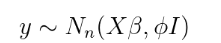到目前为止，这与多元正态回归相同。 则将概率最大化可得出以下解 ：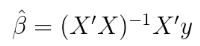贝叶斯模型是通过指定为一个先验分布得到 。在此示例中，我将在以下情况下使用 先验值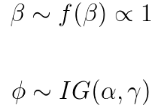block Gibbs

在对采样器进行编码之前，我们需要导出Gibbs采样器的 每个参数的后验条件分布。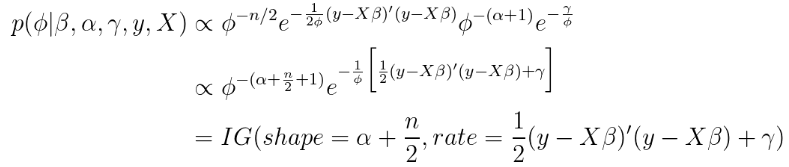条件后验$\ beta$取更多的线性代数。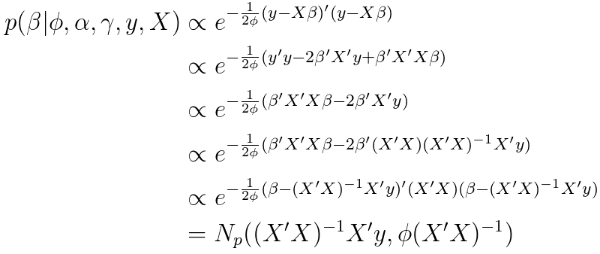这是一个非常漂亮和直观的结果。 条件后验的协方差矩阵是协方差矩阵的估计，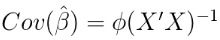还要注意，条件后验是一个多元分布。因此，在Gibbs采样器的每次迭代中，我们从后验绘制出一个完整的矢量 。

模拟

我模拟的 结果向量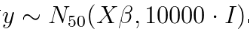。

运行 Gibbs采样器 会生成对真实系数和方差参数的估计。运行了500,000次迭代。周期为100,000次，10次迭代。

以下是MCMC链的图，其中真实值用红线表示。

# 计算后验摘要统计信息
post_dist %>%
group_by(para) %>%
summarise(median=median(draw),
lwr=quantile(.025),
upr=quantile(.975)) %>%

# 合并汇总统计信息
post_dist <- post_dist %>%
left_join(post_sum_stats, by='param')

# 绘制MCMC链
ggplot(post_dist,aes(x=iter,y=dra)) +
geom_line() +
geom_hline(aes(yintercept=true_vals))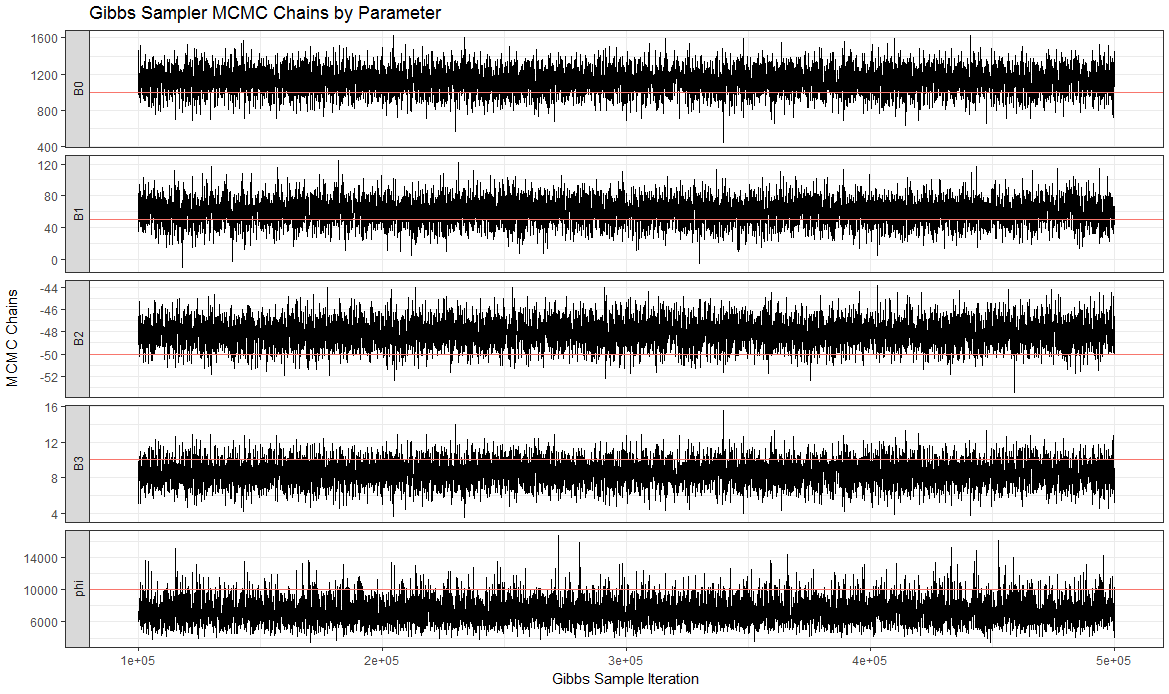这是修整后参数的后验分布：

ggplot(post_dist,aes(x=draw)) +
geom_histogram(aes(x=draw),bins=50) +
geom_vline(aes(xintercept = true_vals))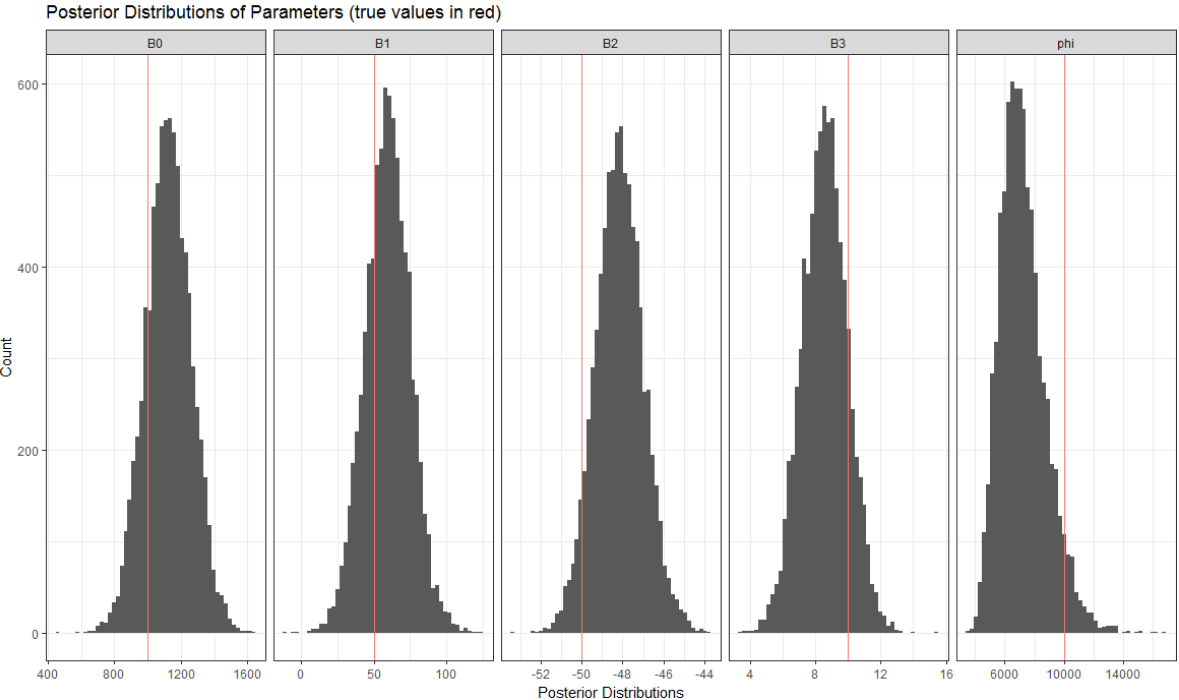似乎能够获得这些参数的合理后验估计。 为了确保贝叶斯估计器正常工作，我对1,000个模拟数据集重复了此过程。

这将产生1,000组后验均值和1,000组95％置信区间。平均而言，这1000个后验均值应以真实值为中心。平均而言，真实参数值应在95％的时间的置信区间内。

以下是这些评估的摘要。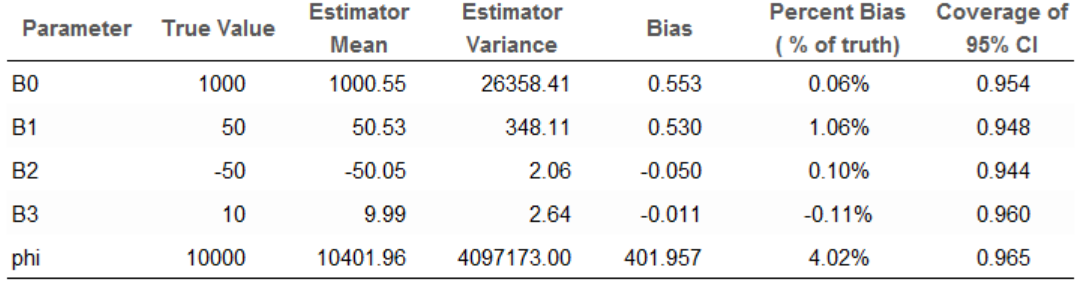“估计平均值”列是所有1,000个模拟中的平均后验平均值。偏差百分比均小于5％。对于所有参数，95％CI的覆盖率约为95％。

扩展

我们可以对该模型进行许多扩展。例如，可以使用除正态分布外的其他分布来拟合不同类型的结果。 例如，如果我们有二元数据，则可以将其建模为：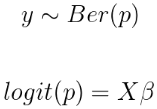然后在上放一个先验分布。这个想法将贝叶斯线性回归推广到贝叶斯GLM。

在本文中概述的线性情况下，可以更灵活地对协方差矩阵建模。相反，假设协方差矩阵是对角线且具有单个公共方差。这是多元线性回归中的同方差假设。如果数据是分类的（例如，每个受试者有多个观察结果），我们可以使用反Wishart分布来建模整个协方差矩阵。最受欢迎的见解

1.matlab使用贝叶斯优化的深度学习

2.matlab贝叶斯隐马尔可夫hmm模型实现

3.R语言Gibbs抽样的贝叶斯简单线性回归仿真

4.R语言中的block Gibbs吉布斯采样贝叶斯多元线性回归

5.R语言中的Stan概率编程MCMC采样的贝叶斯模型

6.Python用PyMC3实现贝叶斯线性回归模型

7.R语言使用贝叶斯 层次模型进行空间数据分析

8.R语言随机搜索变量选择SSVS估计贝叶斯向量自回归（BVAR）模型

9.matlab贝叶斯隐马尔可夫hmm模型实现

展开全文R语言
• 这里给出多元正太分布下的贝叶斯估计的推导过程。（本人比较懒，直接上图趴。。。） 至此推导出了均值与协方差矩阵测估计量；可以与一元正太分布的结果做对比， 发现有很高的相似性！数学之美就在于此嘿嘿嘿~ ...模式识别
• 经典的贝叶多元统计方面的教材，目前大多数网上书店无货，网上又没有免费的资源，因此提供该资源供大家免费共享
• 在这篇文章中，我将对多元线性...贝叶斯多元回归假设该向量是从多元正态分布中得出的，其中均值向量是​和协方差矩阵​。这里​是观察到​的协变量矩阵。注意，该矩阵的第一列是标识。参数矢量​的​， ​是一种常见...
• R包在上，实现了快速统计算法以通过经验贝叶斯解决多元法线均值问题。 快速开始 安装软件包并构建小插图： devtools :: install_github( " stephenslab/udr " , build_vignettes = TRUE ) 请注意，安装该软件包将...R
• 通过贝叶斯模型融合对模拟和混合信号电路进行有效的多元矩估计
• 使用贝叶斯滤波器的时变过程的多元报警系统及其在电动泵上的应用研究论文
• 伯努利贝叶斯：先验为伯努利分布的朴素贝叶斯，适用于样本很稀松的二元离散值或很稀松的多元离散值情况 转载于:https://www.cnblogs.com/liang715200/p/10678032.html...
• 1、贝叶斯分类 1.1、分类规则 和代表两个总体，各自的先验概率为和（），和分别是总体和中的概率密度函数。 和代表按分类规则划分的两组区域。例如，如果一个新观测对象分到，那么我们声明该样本来自总体，。 和 ...
• 目录Part One 理论一、贝叶斯理论简介二、最小错误率贝叶斯决策**三、最小风险贝叶斯决策****Part Two 实操**使用真实数据实战！单特征3特征 这个学期在学模式识别，把常见的算法自己手码出来了，感受颇深，所以给...模式识别 机器学习
• 贝叶斯理论多元正态分布推导-求协方差元素时分子为什么是n-1不是n备忘 参考博客 备忘
• 贝叶斯分为：高斯贝叶斯，多项式贝叶斯，...伯努利贝叶斯：先验为伯努利的朴素贝叶斯，适用于样本特征是二元离散值或者很稀疏的多元离散值情况 import pandas as pd import numpy as np import matplotlib....
• 在本文中，我们通过一个名为WinBUGS的免费贝叶斯软件，可以很容易地完成基于似然的多变量随机波动率（SV）模型的估计和比较。通过拟合每周汇率的双变量时间序列数据，多变量SV模型，包括波动率中的格兰杰因果关系，...WinBUGS
• 先来来看看随机连续分布下贝叶斯分类器模型。假设当前要使用贝叶斯分类器进行二分类的判别，数据集有随机分布，图像如下： ...然后代入多元高斯分布下的条件概率密度函数，m是数据维度（特征数量）。此处只针对二分
• brms程序包提供了一个接口，以使用Stan来拟合贝叶斯广义（非）线性多元多级模型，这是一个用于执行完整贝叶斯推理的C ++程序包（请参见 ）。 公式语法与软件包lme4的语法非常相似，以提供用于执行回归分析的熟悉且...
• 简述多元正态分布下的最小错误率贝叶斯如果特征的值向量服从d元正态分布，即其概率密度函数为： 即其分布可以由均值向量和对称的协方差矩阵 唯一确定。如果认为样本的特征向量在类内服从多元正态分布： 即...机器学习 极大似然估计
• 在本文中，我们将分析横断面工资数据，以期在实践中使用贝叶斯方法，如BIC和贝叶斯模型来构建工资的预测模型。 加载包 在本实验中，我们将使用dplyr包探索数据，并使用ggplot2包进行数据可视化。我们也可以在其中...R语言 线性回归
• 文章目录前言贝叶斯定理贝叶斯优化算法概率代理模型采集函数论文中使用的BO算法概率代理模型：高斯过程采集函数：UCB小结 前言 如有错误，欢迎交流指正。 贝叶斯优化是一种十分有效的全局优化算法,目标是找到全局最...算法 神经网络 机器学习
• 贝叶斯判别，模式识别作业。比较简单。可以参考一下，提供借鉴。...